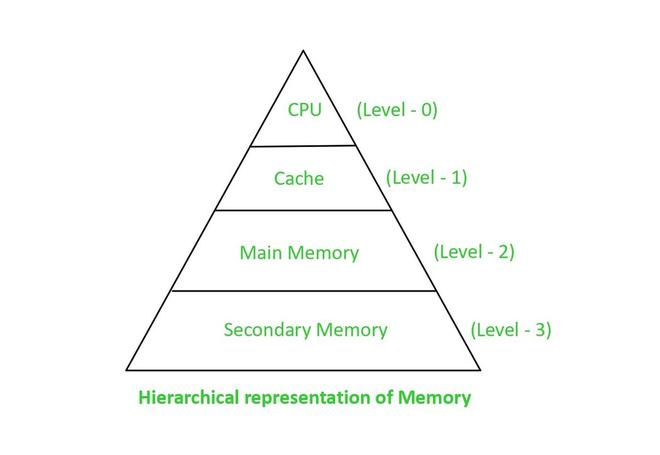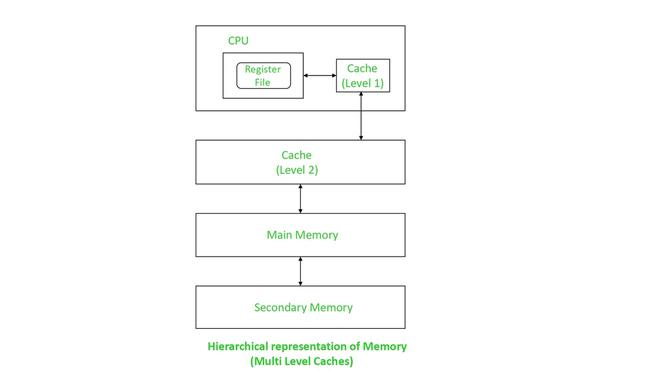Open in App
Not now

# Basic Cache Optimization Techniques

• Difficulty Level : Basic
• Last Updated : 24 Feb, 2023

Generally, in any device, memories that are large(in terms of capacity), fast and affordable are preferred. But all three qualities can’t be achieved at the same time. The cost of the memory depends on its speed and capacity. With the Hierarchical Memory System, all three can be achieved simultaneously.Memory Hierarchy

The cache is a part of the hierarchy present next to the CPU. It is used in storing the frequently used data and instructions. It is generally very costly i.e., the larger the cache memory, the higher the cost. Hence, it is used in smaller capacities to minimize costs. To make up for its less capacity, it must be ensured that it is used to its full potential.

Optimization of cache performance ensures that it is utilized in a very efficient manner to its full potential.

### Average Memory Access Time(AMAT):

AMAT helps in analyzing the Cache memory and its performance. The lesser the AMAT, the better the performance is. AMAT can be calculated as,

```For simultaneous access:
AMAT = Hit Ratio * Cache access time + Miss Ratio * Main memory access time
= (h * tc) + (1-h) * tm
For hierarchial access:
AMAT = Hit Ratio * Cache access time + Miss Ratio * (Cache access time + Main memory access time)
= (h * tc) + (1-h) * (tc+tm)```

Note: Main memory is accessed only when a cache miss occurs. Hence, cache time is also included in the main memory access time.

Example 1: What is the average memory access time for a machine with a cache hit rate of 75% and cache access time of 3 ns and main memory access time of 110 ns.

Solution:

```Average Memory Access Time(AMAT) =  (h * tc) + (1-h) * (tc  + tm)
Given,
Hit Ratio(h) = 75/100 = 3/4 = 0.75
Miss Ratio (1-h) = 1-0.75 = 0.25
Cache access time(tc) = 3ns

Main memory access time(effectively) = tc  +  tm = 3 + 110 = 113 ns
Average Memory Access Time(AMAT) = (0.75 * 3) + (0.25 * (3+110))
=  2.25 + 28.25
=  30.5 ns```
`Note: AMAT can also be calculated as Hit Time + (Miss Rate * Miss Penalty)`

Example 2:  Calculate AMAT when Hit Time is 0.9 ns, Miss Rate is 0.04, and Miss Penalty is 80 ns.

Solution :

```Average Memory Access Time(AMAT) =  Hit Time + (Miss Rate * Miss Penalty)
Here, Given,
Hit time = 0.9 ns
Miss Rate = 0.04
Miss Penalty = 80 ns
Average Memory Access Time(AMAT) = 0.9 + (0.04*80)
= 0.9 + 3.2
= 4.1 ns```

Hence, if Hit time, Miss Rate, and Miss Penalty are reduced, the AMAT reduces which in turn ensures optimal performance of the cache.

### Methods to reduce Hit Time:

1. Small and simple caches: If lesser hardware is required for the implementation of caches, then it decreases the Hit time because of the shorter critical path through the Hardware.

2. Avoid Address translation during indexing: Caches that use physical addresses for indexing are known as a physical cache. Caches that use the virtual addresses for indexing are known as virtual cache. Address translation can be avoided by using virtual caches. Hence, they help in reducing the hit time.

### Methods to reduce Miss Rate:

1. Larger block size:  If the block size is increased, spatial locality can be exploited in an efficient way which results in a reduction of miss rates. But it may result in an increase in miss penalties. The size can’t be extended beyond a certain point since it affects negatively the point of increasing miss rate. Because larger block size implies a lesser number of blocks which results in increased conflict misses.

2. Larger cache size: Increasing the cache size results in a decrease of capacity misses, thereby decreasing the miss rate. But, they increase the hit time and power consumption.

3. Higher associativity: Higher associativity results in a decrease in conflict misses. Thereby, it helps in reducing the miss rate.

### Methods to reduce Miss Penalty:

1. Multi-Level Caches: If there is only one level of cache, then we need to decide between keeping the cache size small in order to reduce the hit time or making it larger so that the miss rate can be reduced. Both of them can be achieved simultaneously by introducing cache at the next levels.

Suppose, if a two-level cache is considered:

• The first level cache is smaller in size and has faster clock cycles comparable to that of the CPU.
• Second-level cache is larger than the first-level cache but has faster clock cycles compared to that of main memory. This large size helps in avoiding much access going to the main memory. Thereby, it also helps in reducing the miss penalty.Hierarchical representation of Memory

2. Critical word first and Early Restart: Generally, the processor requires one word of the block at a time. So, there is no need of waiting until the full block is loaded before sending the requested word. This is achieved using:

• The critical word first: It is also called a requested word first. In this method, the exact word required is requested from the memory and as soon as it arrives, it is sent to the processor. In this way, two things are achieved, the processor continues execution, and the other words in the block are read at the same time.
• Early Restart: In this method, the words are fetched in the normal order. When the requested word arrives, it is immediately sent to the processor which continues execution with the requested word.

These are the basic methods through which the performance of cache can be optimized.

My Personal Notes arrow_drop_up
Related Articles